# 6.4: Assessing Normality

$$\newcommand{\vecs}{\overset { \rightharpoonup} {\mathbf{#1}} }$$ $$\newcommand{\vecd}{\overset{-\!-\!\rightharpoonup}{\vphantom{a}\smash {#1}}}$$$$\newcommand{\id}{\mathrm{id}}$$ $$\newcommand{\Span}{\mathrm{span}}$$ $$\newcommand{\kernel}{\mathrm{null}\,}$$ $$\newcommand{\range}{\mathrm{range}\,}$$ $$\newcommand{\RealPart}{\mathrm{Re}}$$ $$\newcommand{\ImaginaryPart}{\mathrm{Im}}$$ $$\newcommand{\Argument}{\mathrm{Arg}}$$ $$\newcommand{\norm}{\| #1 \|}$$ $$\newcommand{\inner}{\langle #1, #2 \rangle}$$ $$\newcommand{\Span}{\mathrm{span}}$$ $$\newcommand{\id}{\mathrm{id}}$$ $$\newcommand{\Span}{\mathrm{span}}$$ $$\newcommand{\kernel}{\mathrm{null}\,}$$ $$\newcommand{\range}{\mathrm{range}\,}$$ $$\newcommand{\RealPart}{\mathrm{Re}}$$ $$\newcommand{\ImaginaryPart}{\mathrm{Im}}$$ $$\newcommand{\Argument}{\mathrm{Arg}}$$ $$\newcommand{\norm}{\| #1 \|}$$ $$\newcommand{\inner}{\langle #1, #2 \rangle}$$ $$\newcommand{\Span}{\mathrm{span}}$$$$\newcommand{\AA}{\unicode[.8,0]{x212B}}$$

The distributions you have seen up to this point have been assumed to be normally distributed, but how do you determine if it is normally distributed. One way is to take a sample and look at the sample to determine if it appears normal. If the sample looks normal, then most likely the population is also. Here are some guidelines that are use to help make that determination.

1. Histogram: Make a histogram. For a normal distribution, the histogram should be roughly bell-shaped. For small samples, this is not very accurate, and another method is needed. A distribution may not look normally distributed from the histogram, but it still may be normally distributed.
2. Outliers: For a normal distribution, there should not be more than one outlier. One way to check for outliers is to use a modified box plot. Outliers are values that are shown as dots outside of the rest of the values. If you don’t have a modified box plot, outliers are those data values that are:
Above Q3, the third quartile, by an amount greater than 1.5 times the interquartile range (IQR)
Below Q1, the first quartile, by an amount greater than 1.5 times the interquartile range (IQR)

Note

If there is one outlier, that outlier could have a dramatic effect on the results especially if it is an extreme outlier. However, there are times where a distribution has more than one outlier, but it is still normally distributed. The guideline of only one outlier is just a guideline.

3. Normal quantile plot (or normal probability plot): This plot is provided through statistical software on a computer or graphing calculator. If the points lie close to a line, the data comes from a distribution that is approximately normal. If the points do not lie close to a line or they show a pattern that is not a line, the data are likely to come from a distribution that is not normally distributed.

### To create a histogram on the TI-83/84:

1. Go into the STAT menu, and then Chose 1: EditFigure $$\PageIndex{1}$$: STAT Menu on TI-83/84
2. Type your data values into L1.
3. Now click STAT PLOT ($$2^{\text { nd }} Y=$$).Figure $$\PageIndex{2}$$: STAT PLOT Menu on TI-83/84
4. Use 1:Plot1. Press ENTER.Figure $$\PageIndex{3}$$: Plot1 Menu on TI-83/84
5. You will see a new window. The first thing you want to do is turn the plot on. At this point you should be on On, just press ENTER. It will make On dark.
6. Now arrow down to Type: and arrow right to the graph that looks like a histogram (3rd one from the left in the top row).
7. Now arrow down to Xlist. Make sure this says L1. If it doesn’t, then put L1 there (2nd number 1). Freq: should be a 1.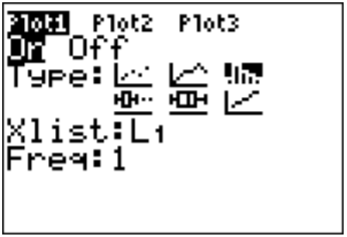Figure $$\PageIndex{4}$$: Plot1 Menu on TI-83/84 Setup for Histogram
8. Now you need to set up the correct window to graph on. Click on WINDOW. You need to set up the settings for the x variable. Xmin should be your smallest data value. Xmax should just be a value sufficiently above your highest data value, but not too high. Xscl is your class width that you calculated. Ymin should be 0 and Ymax should be above what you think the highest frequency is going to be. You can always change this if you need to. Yscl is just how often you would like to see a tick mark on the y-axis.
9. Now press GRAPH. You will see a histogram.

### To find the IQR and create a box plot on the TI-83/84:

1. Go into the STAT menu, and then Choose 1:EditFigure $$\PageIndex{5}$$: STAT Menu on TI-83/84
2. Type your data values into L1. If L1 has data in it, arrow up to the name L1, click CLEAR and then press ENTER. The column will now be cleared and you can type the data in.
3. Go into the STAT menu, move over to CALC and choose 1-Var Stats. Press ENTER, then type L1 (2nd 1) and then ENTER. This will give you the summary statistics. If you press the down arrow, you will see the five-number summary.
4. To draw the box plot press 2nd STAT PLOT.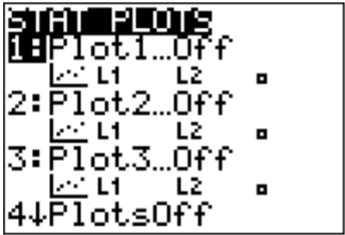Figure $$\PageIndex{6}$$: STAT PLOT Menu on TI-83/84
5. Use Plot1. Press ENTER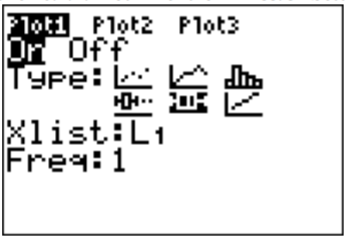Figure $$\PageIndex{7}$$: Plot1 Menu on TI-83/84 Setup for Box Plot
6. Put the cursor on On and press Enter to turn the plot on. Use the down arrow and the right arrow to highlight the boxplot in the middle of the second row of types then press ENTER. Set Data List to L1 (it might already say that) and leave Freq as 1.
7. Now tell the calculator the set up for the units on the x-axis so you can see the whole plot. The calculator will do it automatically if you press ZOOM, which is in the middle of the top row.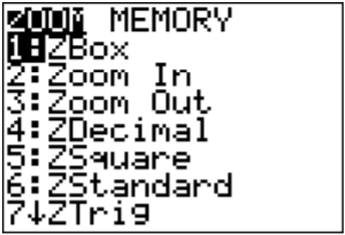Figure $$\PageIndex{8}$$: ZOOM Menu on TI-83/84
Then use the down arrow to get to 9:ZoomStat and press ENTER. The box plot will be drawn.Figure $$\PageIndex{9}$$: ZOOM Menu on TI-83/84 with ZoomStat

### To create a normal quantile plot on the TI-83/84

1. Go into the STAT menu, and then Chose 1:EditFigure $$\PageIndex{10}$$: STAT Menu on TI-83/84
2. Type your data values into L1. If L1 has data in it, arrow up to the name L1, click CLEAR and then press ENTER. The column will now be cleared and you can type the data in.
3. Now click STAT PLOT ($$2^{\text { nd }} Y=$$). You have three stat plots to choose from.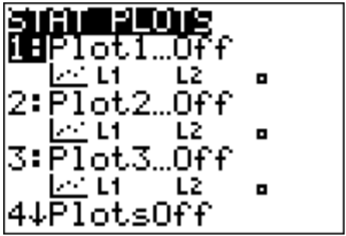Figure $$\PageIndex{11}$$: STAT PLOT Menu on TI-83/84
4. Use 1:Plot1. Press ENTER.
5. Put the cursor on the word On and press ENTER. This turns on the plot. Arrow down to Type: and use the right arrow to move over to the last graph (it looks like an increasing linear graph). Set Data List to L1 (it might already say that) and set Data Axis to Y. The Mark is up to you.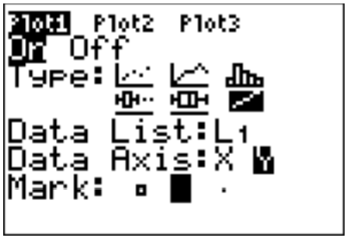Figure $$\PageIndex{12}$$: Plot1 Menu on TI-83/84 Setup for Normal Quantile Plot
6. Now you need to set up the correct window on which to graph. Click on WINDOW. You need to set up the settings for the x variable. Xmin should be -4. Xmax should be 4. Xscl should be 1. Ymin and Ymax are based on your data, the Ymin should be below your lowest data value and Ymax should be above your highest data value. Yscl is just how often you would like to see a tick mark on the y-axis.
7. Now press GRAPH. You will see the normal quantile plot.

### To create a histogram on R:

Put the variable in using variable<-c(type in the data with commas between values) using a name for the variable that makes sense for the problem. The command for histogram is hist(variable). You can then copy the histogram into a word processing program. There are options that you can put in for title, and axis labels. See section 2.2 for the commands for those.

### To create a modified boxplot on R:

Put the variable in using variable<-c(type in the data with commas between values) using a name for the variable that makes sense for the problem. The command for box plot is boxplot(variable). You can then copy the box plot into a word processing program. There are options that you can put in for title, horizontal orientation, and axis labels. See section 3.3 for the commands for those.

### To create a normal quantile plot on R:

Put the variable in using variable<-c(type in the data with commas between values) using a name for the variable that makes sense for the problem. The command for normal quantile plot is qqnorm(variable). You can then copy the normal quantile plot into a word processing program.

Realize that your random variable may be normally distributed, even if the sample fails the three tests. However, if the histogram definitely doesn't look symmetric and bell shaped, there are outliers that are very extreme, and the normal probability plot doesn’t look linear, then you can be fairly confident that the data set does not come from a population that is normally distributed.

Example $$\PageIndex{1}$$ is it normal?

In Kiama, NSW, Australia, there is a blowhole. The data in table #6.4.1 are times in seconds between eruptions ("Kiama blowhole eruptions," 2013). Do the data come from a population that is normally distributed?

 83 51 87 60 28 95 8 27 15 10 18 16 29 54 91 8 17 55 10 35 47 77 36 17 21 36 18 40 10 7 34 27 28 56 8 25 68 146 89 18 73 69 9 37 10 82 29 8 60 61 61 18 169 25 8 26 11 83 11 42 17 14 9 12
1. State the random variable
2. Draw a histogram.
3. Find the number of outliers.
4. Draw the normal quantile plot.
5. Do the data come from a population that is normally distributed?

Solution

a. x = time in seconds between eruptions of Kiama Blowhole

b. The histogram produced is in Figure $$\PageIndex{13}$$.Figure $$\PageIndex{13}$$: Histogram for Kiama Blowhole

This looks skewed right and not symmetric.

c. The box plot is in Figure $$\PageIndex{14}$$.Figure $$\PageIndex{14}$$: Modified Box Plot from TI-83/83 for Kiama Blowhole

There are two outliers. Instead using:

$$I Q R=Q 3-Q 1=60-14.5=45.5$$ seconds

$$1.5 * I Q R=1.5 * 45.5=68.25$$ seconds

$$Q 1-1.5^{*} I Q R=14.5-68.25=-53.75$$ seconds

$$Q 3+1.5 * I Q R=60+68.25=128.25$$ seconds

Outliers are any numbers greater than 128.25 seconds and less than -53.75 seconds. Since all the numbers are measurements of time, then no data values are less than 0 or seconds for that matter. There are two numbers that are larger than 128.25 seconds, so there are two outliers. Two outliers are not real indications that the sample does not come from a normal distribution, but the fact that both are well above 128.25 seconds is an indication of an issue.

d. The normal quantile plot is in Figure $$\PageIndex{15}$$.Figure $$\PageIndex{15}$$: Normal Probability Plot

This graph looks more like an exponential growth than linear.

e. Considering the histogram is skewed right, there are two extreme outliers, and the normal probability plot does not look linear, then the conclusion is that this sample is not from a population that is normally distributed.

Example $$\PageIndex{2}$$ is it normal?

One way to measure intelligence is with an IQ score. Example $$\PageIndex{2}$$ contains 50 IQ scores. Determine if the sample comes from a population that is normally distributed.

 78 92 96 100 67 105 109 75 127 111 93 114 82 100 125 67 94 74 81 98 102 108 81 96 103 91 90 96 86 92 84 92 90 103 115 93 85 116 87 106 85 88 106 104 102 98 116 107 102 89
1. State the random variable.
2. Draw a histogram.
3. Find the number of outliers.
4. Draw the normal quantile plot.
5. Do the data come from a population that is normally distributed?

Solution

a. x = IQ score

b. The histogram is in Figure $$\PageIndex{16}$$.Figure $$\PageIndex{16}$$: Histogram for IQ Score

This looks somewhat symmetric, though it could be thought of as slightly skewed right.

c. The modified box plot is in Figure $$\PageIndex{17}$$.Figure $$\PageIndex{17}$$: Output from TI-83/84 for IQ Score

There are no outliers.

Or using Outliers

$$I Q R=Q 3-Q 1=105-87=18$$

$$1.5^{*} I Q R=1.5^{*} 18=27$$

$$Q 1 -1.5 I Q R=87-27=60$$

$$Q 3+1.5 I Q R=105+27=132$$

are any numbers greater than 132 and less than 60. Since the maximum number is 127 and the minimum is 67, there are no outliers.

d. The normal quantile plot is in Figure $$\PageIndex{18}$$.Figure $$\PageIndex{18}$$: Normal Quantile Plot

This graph looks fairly linear.

e. Considering the histogram is somewhat symmetric, there are no outliers, and the normal probability plot looks linear, then the conclusion is that this sample is from a population that is normally distributed.

## Homework

Exercise $$\PageIndex{1}$$

1. Cholesterol data was collected on patients four days after having a heart attack. The data is in Example $$\PageIndex{3}$$. Determine if the data is from a population that is normally distributed.
 218 234 214 116 200 276 146 182 238 288 190 236 244 258 240 294 220 200 220 186 352 202 218 248 278 248 270 242
2. The size of fish is very important to commercial fishing. A study conducted in 2012 collected the lengths of Atlantic cod caught in nets in Karlskrona (Ovegard, Berndt & Lunneryd, 2012). Data based on information from the study is in Example $$\PageIndex{4}$$. Determine if the data is from a population that is normally distributed.
 48 50 50 55 53 50 49 52 61 48 45 47 53 46 50 48 42 44 50 60 54 48 50 49 53 48 52 56 46 46 47 48 48 49 52 47 51 48 45 47
3. The WHO MONICA Project collected blood pressure data for people in China (Kuulasmaa, Hense & Tolonen, 1998). Data based on information from the study is in Example $$\PageIndex{5}$$. Determine if the data is from a population that is normally distributed.
 114 141 154 137 131 132 133 156 119 138 86 122 112 114 177 128 137 140 171 129 127 104 97 135 107 136 118 92 182 150 142 97 140 106 76 115 119 125 162 80 138 124 132 143 119
4. Annual rainfalls for Sydney, Australia are given in Example $$\PageIndex{6}$$. ("Annual maximums of," 2013). Can you assume rainfall is normally distributed?
 146.8 383 90.9 178.1 267.5 95.5 156.5 180 90.9 139.7 200.2 171.7 187.2 184.9 70.1 58 84.1 55.6 133.1 271.8 135.9 71.9 99.4 110.6 47.5 97.8 122.7 58.4 154.4 173.7 118.8 88 84.6 171.5 254.3 185.9 137.2 138.9 96.2 85 45.2 74.7 264.9 113.8 133.4 68.1 156.4